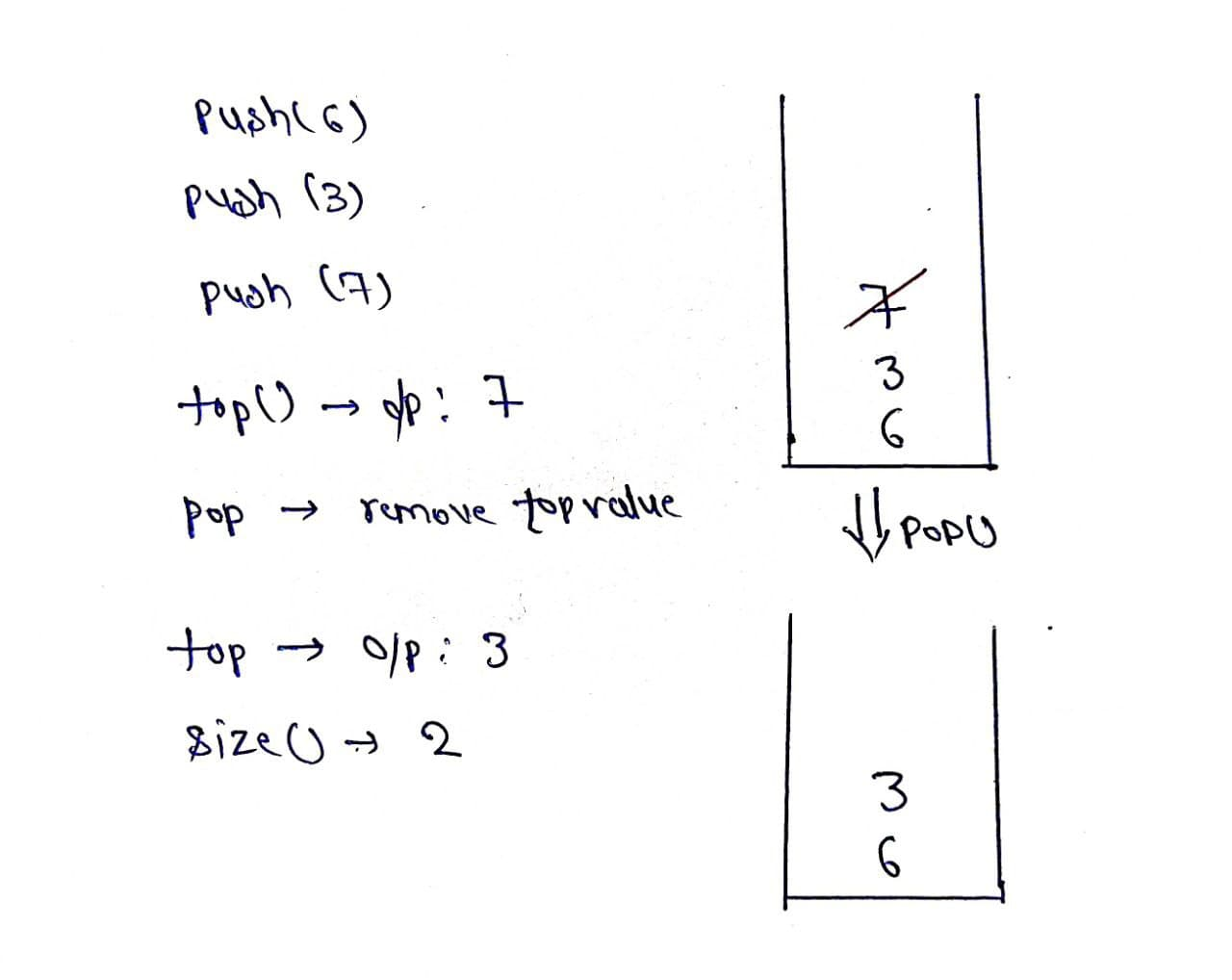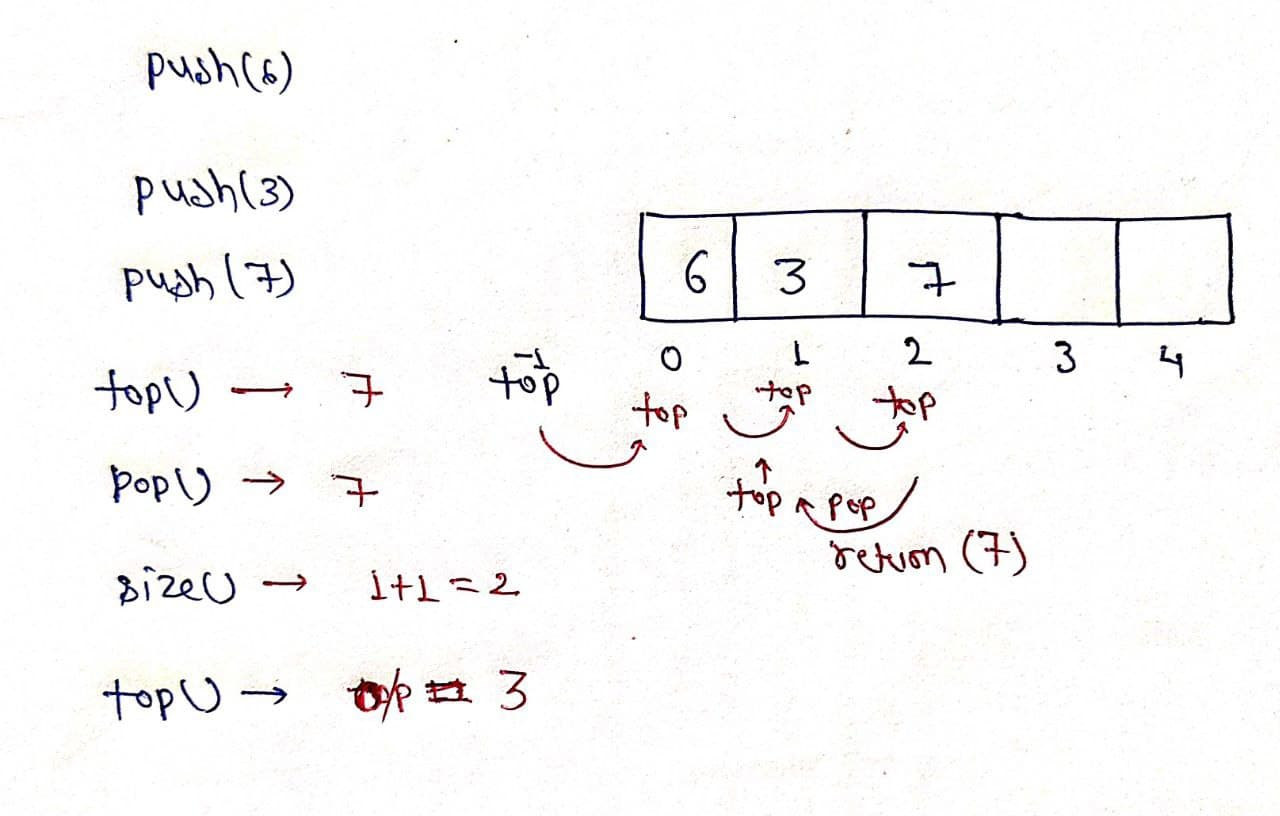# Implement Stack using Array

Problem statement: Implement a stack using an array.

Note: Stack is a data structure that follows the Last In First Out (LIFO) rule.

Example:Explanation

push(): Insert the element in the stack.

pop(): Remove and return the topmost element of the stack.

top(): Return the topmost element of the stack

size(): Return the number of remaining elements in the stack.

### Solution

Disclaimer: Don’t jump directly to the solution, try it out yourself first.

Intuition: As we know stack works on the principle of last in first out, so we have to put elements in an array such that it keeps track of the most recently inserted element. Hence we can think of using a Top variable which will help in keeping track of recent elements inserted in the array.

Approach:

• Declare an array of particular size.
• Define a variable “Top” and initialize it as -1.
• push(x): insert element is the array by increasing top by one.
• pop(): check whether top is not equal to -1 if it is so, return top and decrease its value by one.Code:

## C++ Code

``````#include<bits/stdc++.h>

using namespace std;
class Stack {
int size;
int * arr;
int top;
public:
Stack() {
top = -1;
size = 1000;
arr = new int[size];
}
void push(int x) {
top++;
arr[top] = x;
}
int pop() {
int x = arr[top];
top--;
return x;
}
int Top() {
return arr[top];
}
int Size() {
}
};
int main() {

Stack s;
s.push(6);
s.push(3);
s.push(7);
cout << "Top of stack is before deleting any element " << s.Top() << endl;
cout << "Size of stack before deleting any element " << s.Size() << endl;
cout << "The element deleted is " << s.pop() << endl;
cout << "Size of stack after deleting an element " << s.Size() << endl;
cout << "Top of stack after deleting an element " << s.Top() << endl;
return 0;
}``````

Output:

Top of stack is before deleting any element 7
Size of stack before deleting any element 3
The element deleted is 7
Size of stack after deleting an element 2
Top of stack after deleting an element 3

Time Complexity: O(N)

Space Complexity: O(N)

## Java Code

``````import java.util.*;

public class tuf {

public static void main(String[] args) {

stack s = new stack();
s.push(6);
s.push(3);
s.push(7);
System.out.println("Top of the stack before deleting any element " + s.top());
System.out.println("Size of the stack before deleting any element " + s.size());
System.out.println("The element deleted is " + s.pop());
System.out.println("Size of the stack after deleting an element " + s.size());
System.out.println("Top of the stack after deleting an element " + s.top());
}
}
class stack {
int size = 10000;
int arr[] = new int[size];
int top = -1;
void push(int x) {
top++;
arr[top] = x;
}
int pop() {
int x = arr[top];
top--;
return x;
}
int top() {
return arr[top];
}
int size() {
}
}``````

Output:

Top of the stack before deleting any element 7
Size of the stack before deleting any element 3
The element deleted is 7
Size of the stack after deleting an element 2
Top of the stack after deleting an element 3

Time Complexity: O(N)

Space Complexity: O(N)